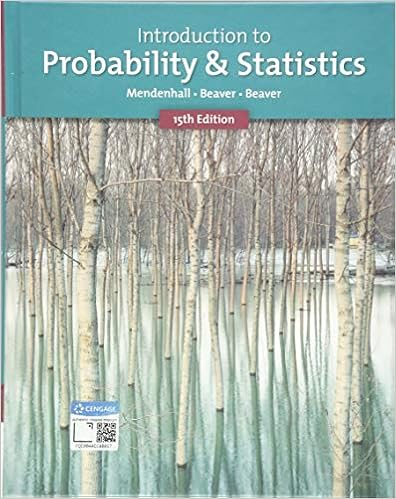# Homework 10 Solutions - Homework Ten Solutions Due December...

• Homework Help
• 5

This preview shows page 1 - 2 out of 5 pages.

##### We have textbook solutions for you!
The document you are viewing contains questions related to this textbook.The document you are viewing contains questions related to this textbook.
Chapter 11 / Exercise 7
Introduction to Probability and Statistics
Beaver/MendenhallExpert Verified
Homework Ten SolutionsDue December 4, 20131. Create a stem and leaf plot of the following data:19.521.022.821.418.718.114.322.422.019.217.816.417.315.214.4143 4152164173 8181 7192 520210 4220 4 82. The definition of a term used in this course is below. Identify the term associated with eachdefinition.(a) A probability sampling method in which the population is first divided into strata(groups) and a simple random sample is then taken from each stratum.What is a stratified random sample?(b) A summary of data showing the fraction or proportion of data values in each of severalnon-overlapping classes.What is a relative frequency distribution?(c) A graphical device used for presenting data summaries based on a subdivision of acircle into sectors that correspond to the relative frequency for each class.What is a pie chart?(d) A summary of quantitative data showing the number of data values that are less thanor equal to the upper class limit of each class.What is a cumulative frequency distribution? OR What is an ogive?(e) A graphical representation of the relationship between two quantitative variables.What is a scatterplot or scatter diagram?3. Given the following scenario, identify the appropriate graph you’d use:(a) We want to understand the distribution of the returns of the 2,308 stocks listed on theNYSE.
##### We have textbook solutions for you!
The document you are viewing contains questions related to this textbook.The document you are viewing contains questions related to this textbook.
Chapter 11 / Exercise 7
Introduction to Probability and Statistics
Beaver/MendenhallExpert Verified
•••